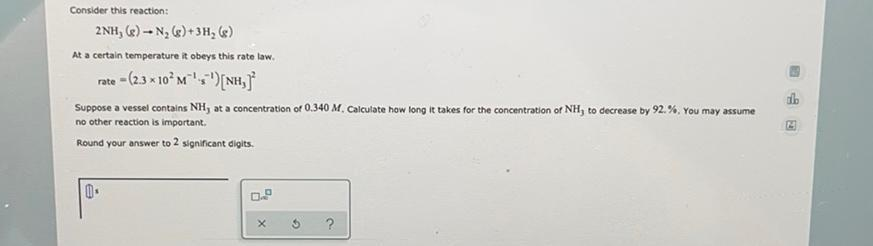Question:

# Consider this reaction: 2NH, (e) - N, ()+3H, () At a certain temperature it obeys this rate law. rate - (2.3 * 10^M^'; ')[NH, SuConsider this reaction: 2NH, (e) - N, ()+3H, () At a certain temperature it obeys this rate law. rate - (2.3 * 10^M^'; ')[NH, Suppose a vessel contains NH, at a concentration of 0.340 M. Calculate how long it takes for the concentration of NH, to decrease by 92.%. You may assume no other reaction is important, Round your answer to 2 significant digits. 0 X 2 ?